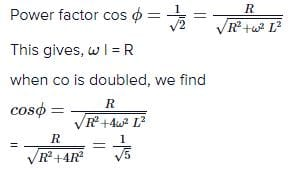NEET  >  Test: Power in an AC Circuit

# Test: Power in an AC Circuit

Test Description

## 10 Questions MCQ Test Physics Class 12 | Test: Power in an AC Circuit

Test: Power in an AC Circuit for NEET 2023 is part of Physics Class 12 preparation. The Test: Power in an AC Circuit questions and answers have been prepared according to the NEET exam syllabus.The Test: Power in an AC Circuit MCQs are made for NEET 2023 Exam. Find important definitions, questions, notes, meanings, examples, exercises, MCQs and online tests for Test: Power in an AC Circuit below.
Solutions of Test: Power in an AC Circuit questions in English are available as part of our Physics Class 12 for NEET & Test: Power in an AC Circuit solutions in Hindi for Physics Class 12 course. Download more important topics, notes, lectures and mock test series for NEET Exam by signing up for free. Attempt Test: Power in an AC Circuit | 10 questions in 10 minutes | Mock test for NEET preparation | Free important questions MCQ to study Physics Class 12 for NEET Exam | Download free PDF with solutions
 1 Crore+ students have signed up on EduRev. Have you?
Test: Power in an AC Circuit - Question 1

### Find the total voltage applied in a series RLC circuit when i=3mA, VL=30V, VC=18V and R=1000 ohms.

Detailed Solution for Test: Power in an AC Circuit - Question 1

Explanation: Total voltage= VR+VL+VC.
VR=1000x3x10-3=3V.
Therefore, total voltage = 30+18+3=51V.

Test: Power in an AC Circuit - Question 2

### The power factor of an RL circuit 1/root2. If the frequency of a.c. is doubled, what will be the power factor?​

Detailed Solution for Test: Power in an AC Circuit - Question 2Test: Power in an AC Circuit - Question 3

### When an emf E = 7cos wt is applied across a circuit, the current is I = 5coswt. What is the power factor for the circuit?

Detailed Solution for Test: Power in an AC Circuit - Question 3

Since E and I are in the same phase.
Therefore, phase difference will be 0 and since power factor= cosx (where x= phase difference) and x =0
therefore, cos x or power factor will be =1

Test: Power in an AC Circuit - Question 4

What is value of power factor if Ø = 90°​

Detailed Solution for Test: Power in an AC Circuit - Question 4

P=Irms Vrms cos ϕ
Where, ϕ=90
cosϕ=0
therefore,P=0
hence the correct answer is option A.

Test: Power in an AC Circuit - Question 5

Power in an ac circuit is equal to

Test: Power in an AC Circuit - Question 6

If the instantaneous current in a circuit is given by i = 2 cos (ωt - φ) A, the rms value of the current is

Detailed Solution for Test: Power in an AC Circuit - Question 6

i=2cost
=Im​cost
So, Im​=2amp
IRMS​=Im​​/√​2​
=2/√​2​
=2​amp

Test: Power in an AC Circuit - Question 7

Find the true power given apparent power = 10 W and power factor = 0.5​

Detailed Solution for Test: Power in an AC Circuit - Question 7

The formula of true power is,
True power=Apparent power x power factor
So, true power=10 x 0.5
True power=5W

Test: Power in an AC Circuit - Question 8

What is the average power/cycle in a capacitor?​

Detailed Solution for Test: Power in an AC Circuit - Question 8

The average power consumed/cycle in an ideal capacitor is 0.
The average power consumed in an ideal capacitor is given as based on the instantaneous power which is supplied to the capacitor:
pc = iv= (im cos ωt)(vm sin ωt)
pc = imvm (cos ωt sin ωt)
pc=(imvm/2)sin2ωt
We know that sin ωt = 0
Therefore, average power = 0

Test: Power in an AC Circuit - Question 9

What is phase angle given R = 10, Z = 20​

Detailed Solution for Test: Power in an AC Circuit - Question 9

Cos x (power factor) = R/Z
= 10/20
=1/2
x= 60

Test: Power in an AC Circuit - Question 10

In a circuit, the reactance X and resistance R are equal. What is the power factor?​

Detailed Solution for Test: Power in an AC Circuit - Question 10

Given that,
Reactance (X) =Resistance (R)
tanϕ = X/R​
So, we have ϕ = π/4​
Power factor = cosϕ = ​1​/√2

## Physics Class 12

157 videos|452 docs|213 tests
 Use Code STAYHOME200 and get INR 200 additional OFF Use Coupon Code
Information about Test: Power in an AC Circuit Page
In this test you can find the Exam questions for Test: Power in an AC Circuit solved & explained in the simplest way possible. Besides giving Questions and answers for Test: Power in an AC Circuit, EduRev gives you an ample number of Online tests for practice

## Physics Class 12

157 videos|452 docs|213 tests

### How to Prepare for NEET

Read our guide to prepare for NEET which is created by Toppers & the best Teachers